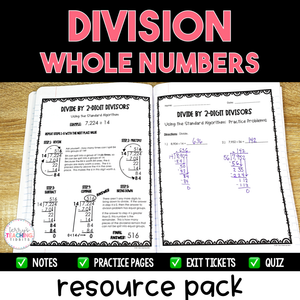# Division of Whole Numbers Resource Pack {Printable}

• \$14.00
Unit price per
Shipping calculated at checkout.

This printable resource pack contains everything you need to teach Division of Whole Numbers:

• detailed notes
• student practice pages
• exit tickets
• quiz.

You will successfully help your students learn how to use mental math, the standard algorithm, and partial quotients to divide by 1-digit and 2-digit divisors.

This resource is also part of my 5th Grade Math Year-Long Curriculum Bundle.

Standard:  5.NBT.6

## What You Get

9 Mini-Lessons

Division with Mental Math

• detailed notes with sample problems
• 3 practice activities
• 3 exit tickets

Division: 1-Digit Divisors {Standard Algorithm}

• 3 mini-lessons
• standard algorithm
• notes with sample problems
• practice activity
• zeros in the quotient
• notes with sample problems
• practice activity
• remainders
• notes with sample problems
• practice activity
• 2 mixed reviews of the 3 types of problems above
• 6 exit tickets

Division: 1-Digit Divisors {Partial Quotients}

• detailed notes with sample problems
• 2 practice pages
• 4 exit tickets

Division: 2-Digit Divisors {Standard Algorithm}

• 3 mini-lessons
• standard algorithm
• notes with sample problems
• practice activity
• zeros in the quotient
• notes with sample problems
• practice activity
• remainders
• notes with sample problems
• practice activity
• 2 mixed reviews of the 3 types of problems above
• 6 exit tickets

Division: 2-Digit Divisors {Partial Quotients}

• detailed notes with sample problems
• 2 practice pages
• 4 exit tickets

Quiz